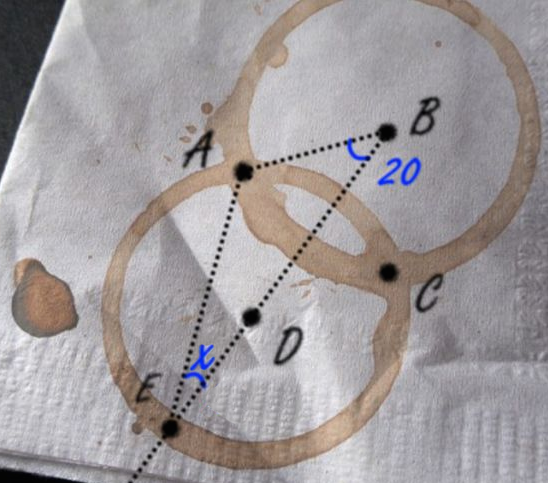# The Geometry Of Coffee Cup Rings

Geometry Level 2Given that $B$ and $D$ are the centers of the two circular prints, what is the value of the angle labeled $x?$

×